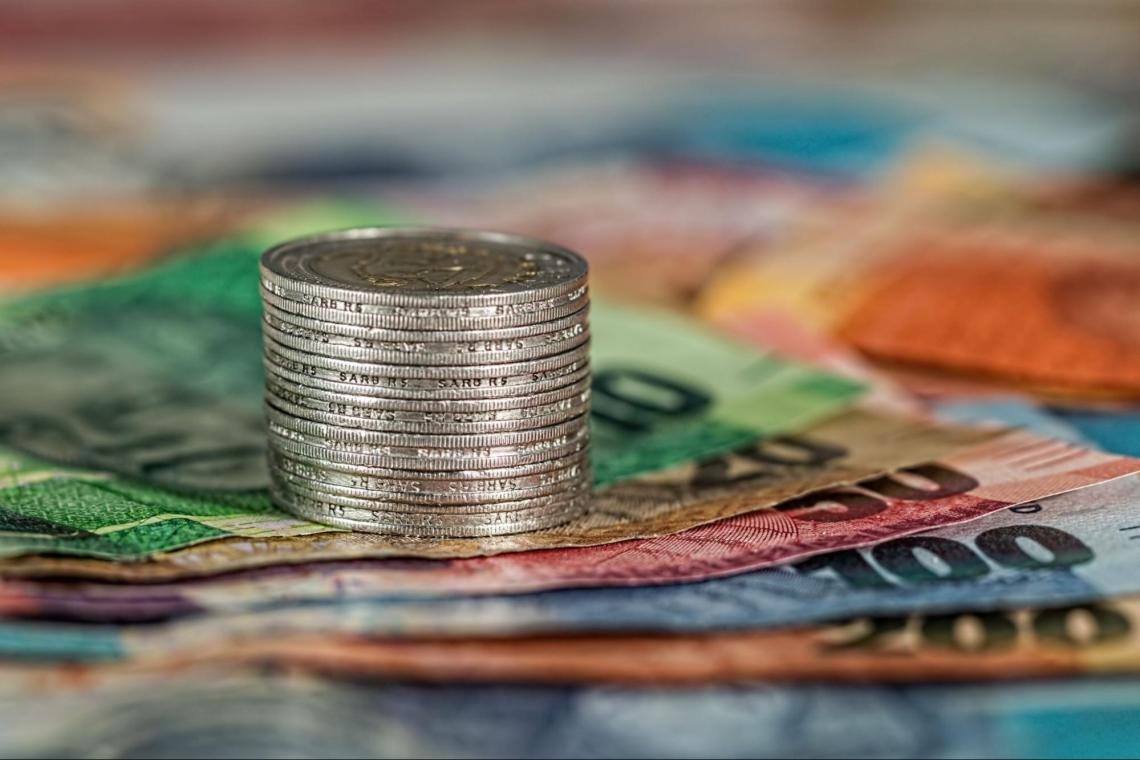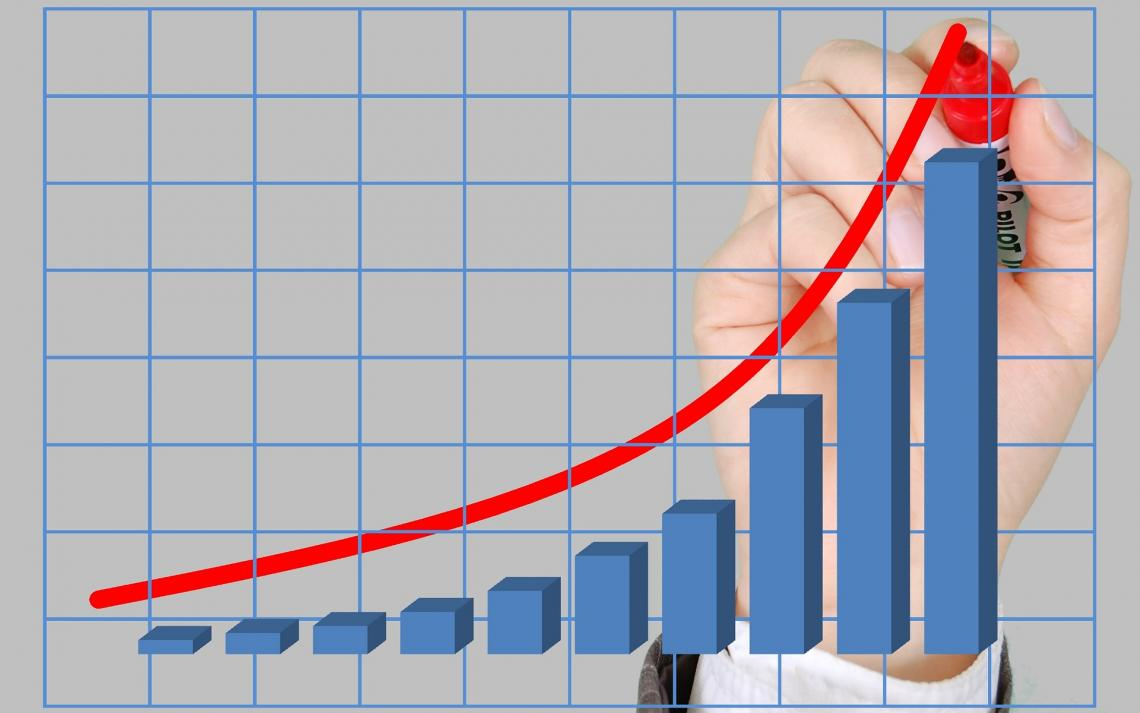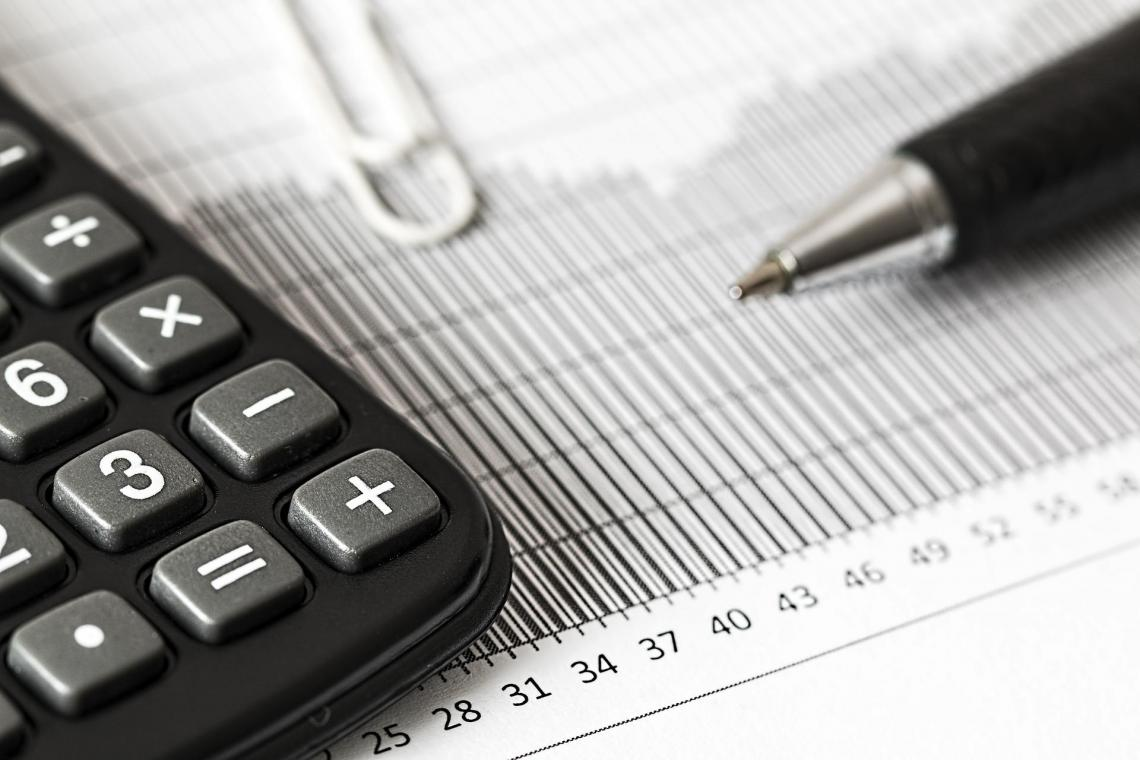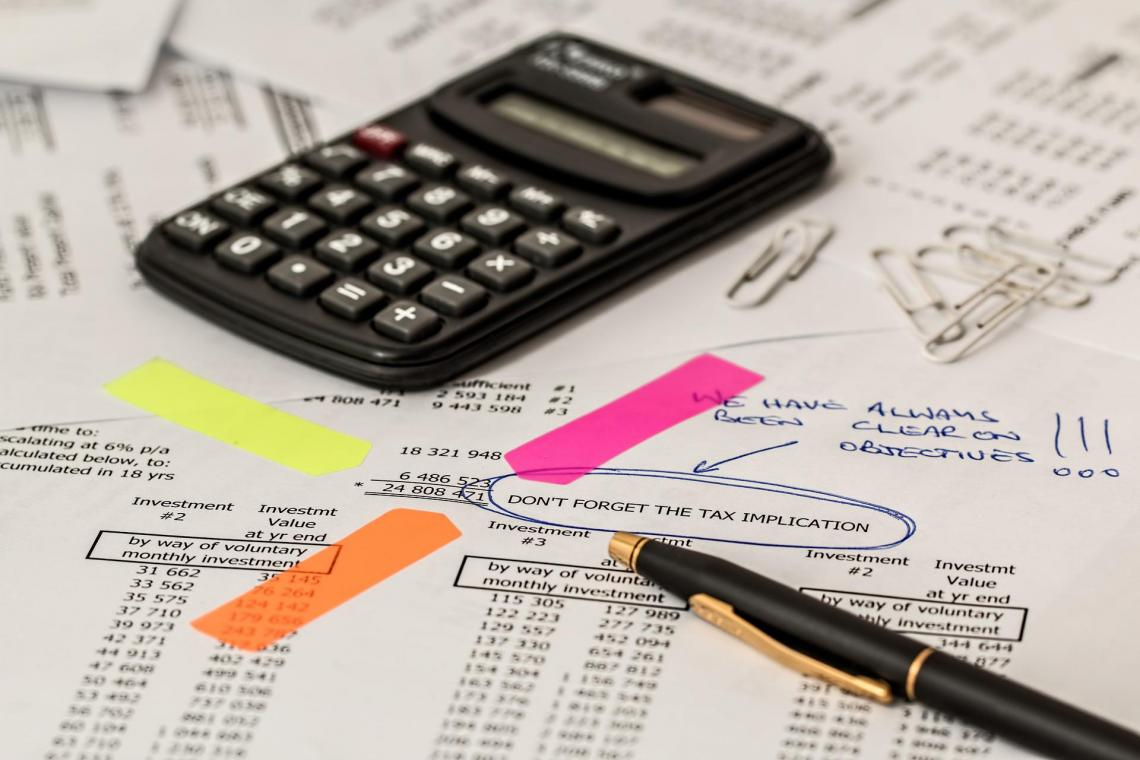# Simple Interest vs Compound Interest

Understanding the two types of interest rates paid by borrowers on the amount borrowed.

Simple interest is the sum of money a borrower pays to a lender on top of the original principal borrowed. The principal is the initial sum of money borrowed or the initial sum deposited/invested.If someone were to borrow money from the bank, they would pay back more than the principal due to interest. The bank is the lender in this case. Borrowing a bank can help, such as financing a large purchase and debt consolidation.

Moreover, if the person deposits money in the bank in savings, the bank will be the borrower, while the depositor will be the lender. Hence, the person depositing money will earn interest from the bank.

A lender (e.g., a person depositing money in the bank) can earn two different types of interest for lending money:

1. Simple interest:  The interest earned only on the initial sum of money that was lent/deposited/invested. This initial sum of money can also be referred to as the principal. Therefore, it is the interest earned only on the principal.

2. Compound interest: The interest earned on both the initial principal invested and the accumulated interest from previous periods. It can be thought of as interest on interest.

The difference between the two lies in the way interest accumulates. Simple interest only rewards interest on the principal, while compound interest rewards interest on both the principal and stacked interest from previous periods.Which type of interest is more beneficial to someone depositing money in the bank? Logically, an account that earns compound interest is more advantageous to the lender than an account that makes simple interest.

Compound interest is more favorable for the lender because the person is earning interest on the principal and the previously acquired interest. In contrast, simple interest only rewards the principal invested, resulting in a lower return.

## simple interest vs. compound interest

The difference between simple and compound interest is the lender receives the way interest:

1. A bank account that offers simple interest pays interest on the deposited amount

2. A bank account that offers compound interest pays interest on the deposited amount and the interest previously rewarded to the depositor.

Most bank accounts offer compound interest. Banks will continue offering compound interest if the interest earned is withdrawn from the account. Therefore, starting a high-yield savings account in a bank offering compounding interest is attractive to low-risk investors.

3. An account with compound interest will have a higher future value than an account that offers simple interest.

A sum of money deposited in a bank account will earn interest each period, meaning that the future value of this sum of money after one period, for instance, will be greater than the current account balance.

In other words, given an equivalent deposit and the same interest on two different accounts, the account with compound interest will have a larger balance than the account with simple interest at any given time.The difference between the balance of the two accounts will tend to increase gradually with every passing period until the difference becomes very apparent because the deposit with simple interest will grow steadily. In contrast, the deposit with compound interest will grow exponentially.

While it is easy to distinguish between the two types of compounding, it is crucial to understand the benefits of each class when it comes to borrowing versus lending money.

## Simple interest and its calculation

As stated, it is called "simple" because it only rewards interest on the principal amount. Assuming a fixed-sized principal, a bank account with such interest will earn the same interest payment each period.Suppose you invested \$2,000 in a bank account that earns 10% interest each year. After one year, the account will have a balance of \$2,200. In other words, the future value of this account in 1 year is \$2,200.

After two years, however, it becomes a bit more complicated to calculate the account's future value because, at the end of year 1, the account had a balance of \$2,200. So, what should that account balance be at the end of the second year?

Since the bank account only pays interest on the principal, the account's future value at the end of the second year must be \$2,400. Interest is only earned on the initial \$2,000 invested in the bank account.

In other words, the account earns equal amounts of interest every period. Each year, \$200 gets added to the balance as interest. The 10% rate is only rewarded on the principle of \$2,000 deposited into the account on day 1.

Therefore, at the end of year 3, the account would have a balance (future value) of \$2,600. At the end of year 4, the account would have a balance of \$2,800, and so on. Yearly interest earned amounts to \$200.

To further simplify the calculations, there is a formula for calculating it on an investment:

Simple Interest = P *i*n

• P is the principal amount (initial amount of investment),
• i is the interest rate (can be annual, semiannual, or monthly interest rate),
• n is the number of periods.The number of periods should be denominated in the same time unit as the interest rate. That is to say, if the interest rate is quoted as a monthly rate, the number of periods must be in months, not years.

If you want to calculate the future value of an investment, add the principal to the simple interest calculated:

FV =(P *i*n)+P

For example, suppose we want to calculate the interest earned on a \$10,000 deposit made to a bank account after five years with an annual interest rate of 7%. In that case, we use the following formula.

• P = \$10,000,
• i = 7% = 0.07,
• n = 5 years (we shouldn't change the years to months or half-years since the interest is quoted as an annual rate)

Hence, simple interest = P * I * N = \$10,000 * 0.07 * 5 = \$3,500. Therefore, the account will earn \$3,500 in interest throughout the five years. At the end of year 3, the account will have a balance (or future value) of \$13,500.

## Compound interest and its calculation

Interest accrued on the principal and the interest from prior periods is compound interest. It is the interest paid on already-earned interest. Almost all banks offer this type of interest on deposits in their bank accounts.Compared to an account that pays interest on the principal deposit, one that compounds interest will have a larger balance in the future because, in contrast, the latter will earn interest on the interest that has already accrued.

Suppose you invested \$2,000 in a bank account that earns 10% interest each year. After one year, the account will have a balance of \$2,200. In other words, the future value of this account in 1 year is \$2,200.

The account has a balance of \$2,200. What should the account balance be at the end of the second year? Since this bank account receives compounding interest, the 10% interest is applied on both the principal and the interest accumulated from year 1.

In other words, the 10% reward is applied to the \$2,200 balance instead of just the initial \$2,000. Therefore, the FV of the account at the end of the second year will be \$2,420. Interest is earned on the initial \$2,000 invested in the account and the \$200 interest.

The future value of the investment at the end of year 2 is \$2,420. Similarly, the 10% interest applies to the \$2,420 included in the bank account. Therefore, the future value of the investment at the end of year 3 is \$2,662.To ease further calculations, there is a formula for calculating the future value of an investment characterized by compound interest:

FV =P (1+i)^n

• P is the principal amount (initial amount of investment)
• i is the periodical interest rate
• n is the number of periods

## Annual, semiannual, quarterly, and monthly compounding

Suppose the interest wasn't quoted as an annual rate but quarterly or monthly. What would happen to the future value of a bank account whose interest is compounded monthly compared to an account with annual compounding?Logically, with two equal deposits in 2 bank accounts that offer the same interest rate, the account with monthly compounding will have a higher future value than the account with yearly compounding.

If an account rewards an interest rate of 10% compounded semiannually, the principal earns 5% interest every six months. However, if the interest were compounded quarterly, the principal would earn 2.5% interest every three months.

Why does an investment with more frequent compounding have a higher future value than an account with less frequent compounding? It is because the account's balance will increase more frequently, and interest will be earned more frequently, yielding a higher return.

The formula for calculating the future value of a principal with multiple rounds of compounding per year is:

FV = PV [1 + in]^nt

• FV is the value of the account or the asset at a later date.
• PV is the current balance of the account (the principal) or the asset's current value.
• i is the interest rate or rate of growth
• n is the number of times the interest is being compounded in a year (n=2 for semiannual compounding, n=4 for quarterly compounding, and n=12 for monthly compounding)
• t is the number of years after which we want to calculate the future value of the account or assetSuppose an account with \$10,000 earns 8% interest, compounded monthly. To calculate the future value of this account in 3 years, we use the formula for frequent compounding:

FV = PV [1 + in]^nt=\$10,000(1+0.0812)12*3= \$12,702.37

Therefore, after three years, the account would have a balance (a future value) of \$12,702.37. Suppose that the same bank account with a \$10,000 principal is currently only offering annual compounding. What will the future value of this account be after three years with a return of 8%?

Before calculating the future value of this investment, it is essential to think critically. Since, in this case, the money in the account is compounded yearly, we should expect its future value to be less than the investment with monthly compounding.

We will use the following formula to calculate the future value of the account:

FV =P (1+i)^n=\$10,000 (1+0.08)3=\$12,597.12### Everything You Need To Build Your Accounting Skills

To Help You Thrive in the Most Flexible Job in the World.

Researched and Authored by Vatche Tchelderian | LinkedIn